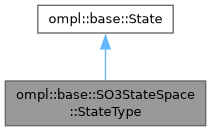ompl::base::SO3StateSpace::StateType Class Reference

The definition of a state in SO(3) represented as a unit quaternion. More...

#include <ompl/base/spaces/SO3StateSpace.h>

Inheritance diagram for ompl::base::SO3StateSpace::StateType:[legend]

## Public Member Functions

void setAxisAngle (double ax, double ay, double az, double angle)
Set the quaternion from axis-angle representation. The angle is given in radians.

void setIdentity ()
Set the state to identity – no rotation.Public Member Functions inherited from ompl::base::State
template<class T >
const T * as () const
Cast this instance to a desired type. More...

template<class T >
T * as ()
Cast this instance to a desired type. More...

## Public Attributes

double x
X component of quaternion vector.

double y
Y component of quaternion vector.

double z
Z component of quaternion vector.

double w
scalar component of quaternion

## Detailed Description

The definition of a state in SO(3) represented as a unit quaternion.

Note
The order of the elements matters in this definition for the SO3StateUniformSampler::sample() function.

Definition at line 90 of file SO3StateSpace.h.

The documentation for this class was generated from the following files: